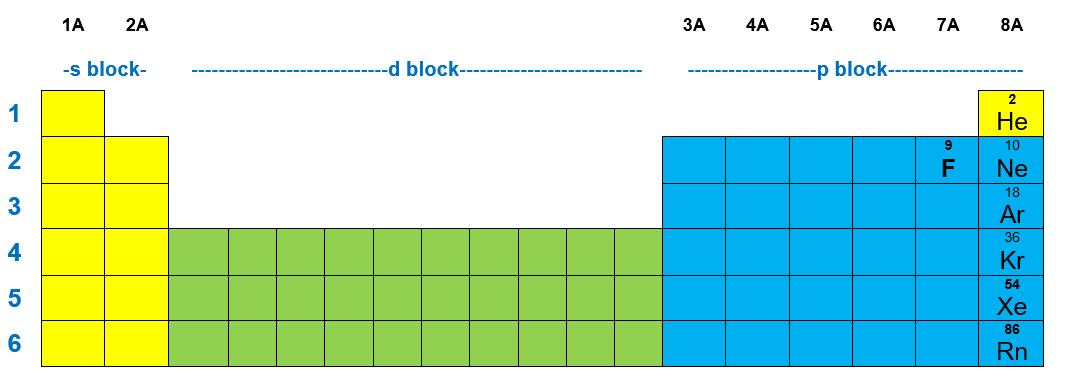# Problem: Imagine a universe in which the value of ms can be +1/2, 0, and -1/2. Assuming that all the other quantum numbers can take only the values possible in our world and that the Pauli exclusion principle applies, give the following.The number of unpaired electrons in fluorine.

###### FREE Expert Solution

We have to determine the number of unpaired electrons in an alternate universe whether the spin quantum number can have three possible values (-½, 0 and +½).

To solve this problem, we will first have to write the electron configuration of fluorine.

Fluorine belongs to the group 7A and has the atomic number 9.Before reaching F, you will pass through the atomic orbitals 1s and 2s.

• In a neutral atom:

Atomic number= # of protons = # of electrons

F: atomic number = 9 → 9 protons & 9 electrons###### Problem Details

Imagine a universe in which the value of ms can be +1/2, 0, and -1/2. Assuming that all the other quantum numbers can take only the values possible in our world and that the Pauli exclusion principle applies, give the following.

The number of unpaired electrons in fluorine.